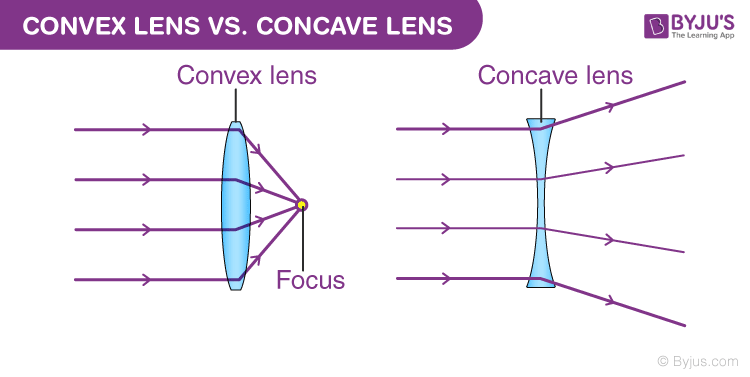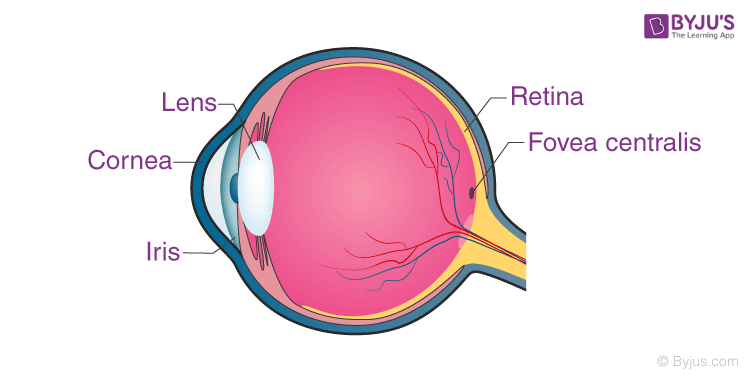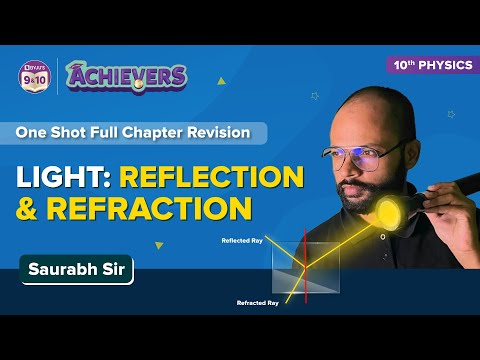# Convex Lens

A lens is a piece of transparent material which concentrates or disperses light rays when passes through them by refraction. According to the shape and purpose of the lens, they are classified into two types convex lens and concave lens. Let’s learn about convex lenses in detail in this article.

## Definition of Convex Lens

This type of lens is thicker at the centre and thinner at the edges.

An optical lens is generally made up of two spherical surfaces. If those surfaces are bent outwards, the lens is called a biconvex lens or simply a convex lens. These types of lenses can converge a beam of light coming from outside and focus it to a point on the other side. This point is known as the focus and the distance between the centre of the lens to the focus is called the focal length of the convex lens. However if one of the surfaces is flat and the other convex, then it is called a plano-convex lens.

## Convex Lens Explained### Difference between Convex and Concave Lens

There is another type of lens known as a concave lens. The major differences between concave and convex lens two are:

CONVEX CONCAVE
This lens converges a straight beam of light. This lens diverges a straight beam of light.
This lens is thicker at the centre and gets thinner as we move towards the edges. This lens is thinner at the centre and gets thicker as we move towards the edges.• A concave lens will always produce a diminished, upright and virtual image of the object in front of it. But the nature of the image produced by a convex lens depends upon the position of the object. It can form both real and virtual images depending on where the light is coming from.

### Uses of Convex Lens

These are used for a variety of purposes in our day-to-day lives. For example,

• The lens in the human eyes is the prime example. So the most common use of the lens is that it helps us to see.• Another common example of the use of this type of lens is a magnifying glass. When an object is placed in front of it at a distance shorter than the focal length of the lens, it produces a magnified and erect image of the object on the same side as the object itself.
• It is used to correct Hypermetropia or long-sightedness.
• It is used in cameras because it focuses light and produces a clear and crisp image.
• More generally these are often used in compound lenses used in various instruments such as magnifying devices like microscopes, telescopes and camera lenses.
• A simple kind of these lenses can focus light into an image, but that image won’t be of high quality. For correcting the distortions and aberrations, it is better to combine both types of lenses.

## Watch the video and revise all the important concepts in the chapter Light Reflection and Refraction Class 10## Frequently Asked Questions – FAQs

Q1

### What are convex lenses made of?

Convex lenses are made of glass or transparent plastic.
Q2

### Can a convex lens produce virtual images?

Convex lenses can form either real or virtual images depending on the position of the object.
Q3

### What is the focal length of a convex lens?

The focal length of a convex lens is the distance between the optical centre and its focus.
Q4

### What are 3 examples of convex lens uses?

Magnifying glasses, cameras and microscopes
Q5

### What are the two basic types of convex lenses?

Biconvex lens and Plano-convex lens

## Recommended Videos

#### Previous year questions in the chapter Light Reflection and Refraction#### Theory and important questions of the chapter Light: Reflection and Refraction